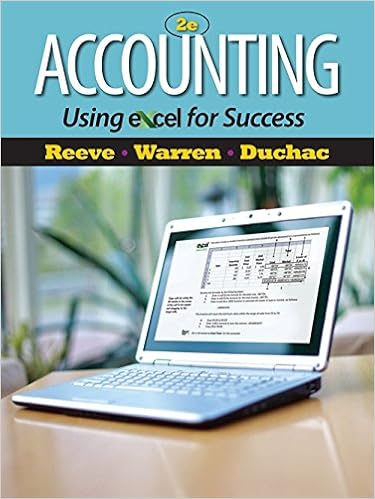# Diff 2 objective 2 aacsb analytical thinking 33

• Test Prep
• 71
• 96% (291) 280 out of 291 people found this document helpful

This preview shows page 26 - 29 out of 71 pages.

##### We have textbook solutions for you!
The document you are viewing contains questions related to this textbook.The document you are viewing contains questions related to this textbook.
Chapter 6 / Exercise 6-5
Accounting Using Excel for Success
Reeve/WarrenExpert Verified
Diff: 2Objective: 2AACSB: Analytical thinking
##### We have textbook solutions for you!
The document you are viewing contains questions related to this textbook.The document you are viewing contains questions related to this textbook.
Chapter 6 / Exercise 6-5
Accounting Using Excel for Success
Reeve/WarrenExpert Verified
3.3 Objective 3.31) Stephanie's Bridal Shoppe sells wedding dresses. The average selling price of each dressis \$1,200, variable costs are \$700, and fixed costs are \$100,000. How many dresses must the Bridal Shoppe sell to yield after-tax net income of \$20,000, assuming the tax rate is 40%?
A
Diff: 3Objective: 3AACSB: Analytical thinking2) Anglico's most recent income statement is given below.Sales (8,000 units)\$160,000Less variable expenses (68,000)Contribution margin 92,000Less fixed expenses (50,000)Net income \$42,000Required:a. Contribution margin per unit is \$ ________ per unitb. If sales are doubled total variable costs will equal \$ ________c. If sales are doubled total fixed costs will equal \$ ________d. If 20 more units are sold, profits will increase by \$ ________e. Compute how many units must be sold to break even. # ________f. Compute how many units must be sold to achieve operating income of \$60,000.# ________g. Compute the revenue needed to achieve an after taxincome of \$30,000 given a tax rate of 30%. \$ ________
Diff: 3Objective: 3AACSB: Application of knowledge3) Black Pearl, Inc., sells a single product. The company's most recent income statement is given below.Sales\$50,000Less variable expenses(30,000)Contribution margin20,000Less fixed expenses(12,500)Net income\$ 7,500Required:a.Contribution margin ratio is________%b.Breakeven point in total sales dollars is\$ ________ c.To achieve \$40,000 in operating income, sales must total\$ ________ d.If sales increase by \$50,000, operating income will increaseby\$ ________ e.To achieve a \$40,000 after tax income, given a tax rate of20%, sales must total\$________Answer:
Diff: 3Objective: 3AACSB: Application of knowledge
•••